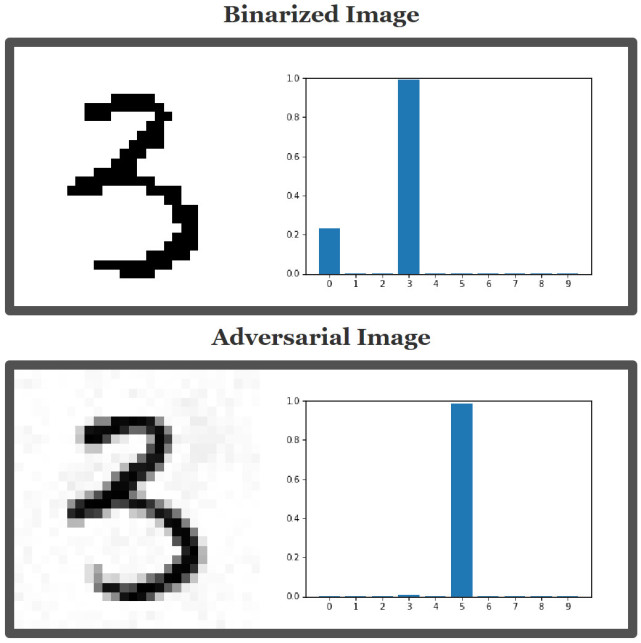# 脆弱的神经网络：UC Berkeley详解对抗样本生成机制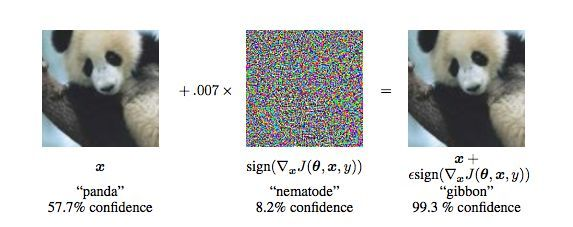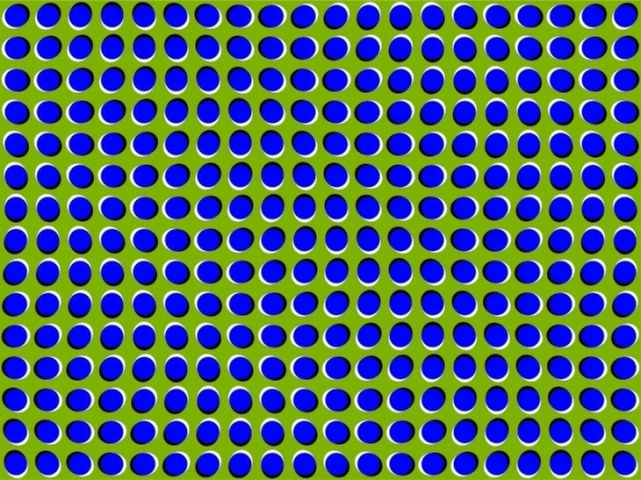MNIST 中的对抗样本6 张 MNIST 图像并排摆放

```import network.network as network

import pickle

import matplotlib.pyplot as plt

import numpy as np

```

```with open('trained_network.pkl', 'rb') as f:

```

```def predict(n):

# Get the data from the test set

x = test_data[n]

# Get output of network and prediction

activations = net.feedforward(x)

prediction = np.argmax(activations)

# Print the prediction of the network

print('Network output: ')

print(activations)

print('Network prediction: ')

print(prediction)

print('Actual image: ')

# Draw the image

plt.imshow(x.reshape((28,28)), cmap='Greys')

```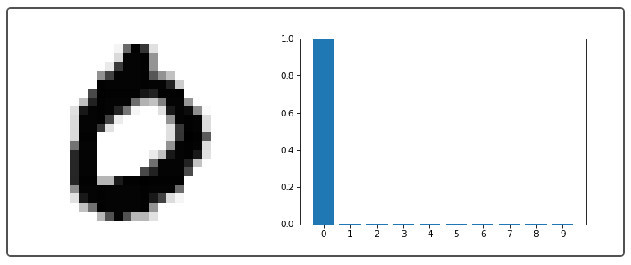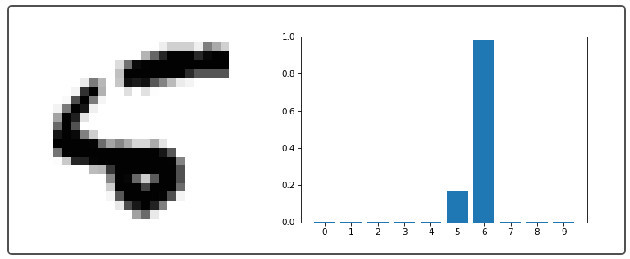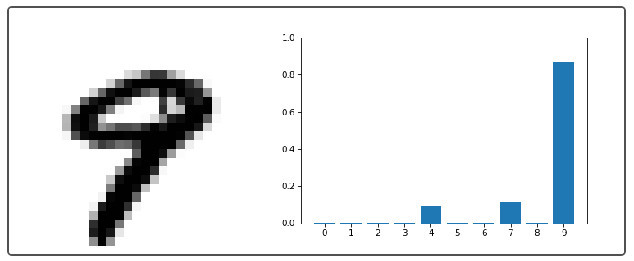```
def adversarial(net, n, steps, eta): """ net : network object     neural network instance to use n : integer     our goal label (just an int, the function transforms it into a one-hot vector) steps : integer     number of steps for gradient descent eta : integer     step size for gradient descent """ # Set the goal output goal = np.zeros((10, 1)) goal[n] = 1 # Create a random image to initialize gradient descent with x = np.random.normal(.5, .3, (784, 1)) # Gradient descent on the input for i in range(steps):     # Calculate the derivative     d = input_derivative(net,x,goal)     # The GD update on x     x -= eta * d return x

```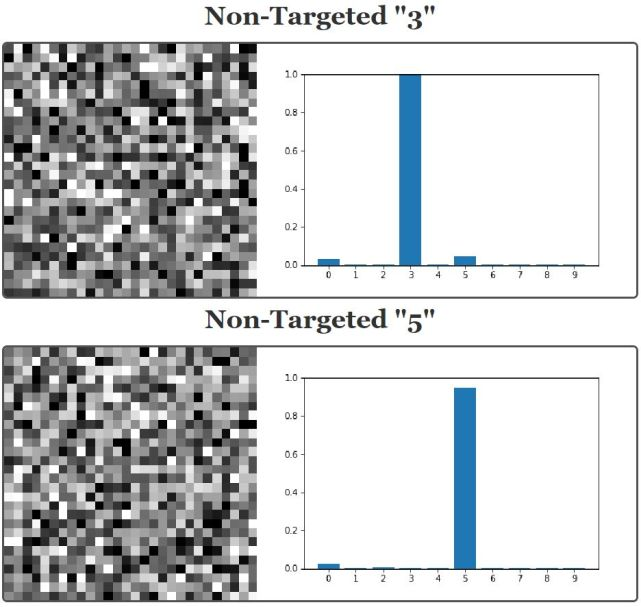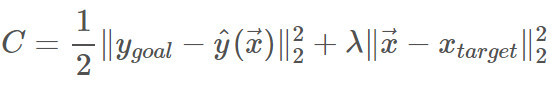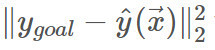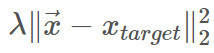```
def sneaky_adversarial(net, n, x_target, steps, eta, lam=.05): """ net : network object     neural network instance to use n : integer     our goal label (just an int, the function transforms it into a one-hot vector) x_target : numpy vector     our goal image for the adversarial example steps : integer     number of steps for gradient descent eta : integer     step size for gradient descent lam : float     lambda, our regularization parameter. Default is .05 """ # Set the goal output goal = np.zeros((10, 1)) goal[n] = 1 # Create a random image to initialize gradient descent with x = np.random.normal(.5, .3, (784, 1)) # Gradient descent on the input for i in range(steps):     # Calculate the derivative     d = input_derivative(net,x,goal)     # The GD update on x, with an added penalty     # to the cost function     # ONLY CHANGE IS RIGHT HERE!!!     x -= eta * (d + lam * (x - x_target)) return x

```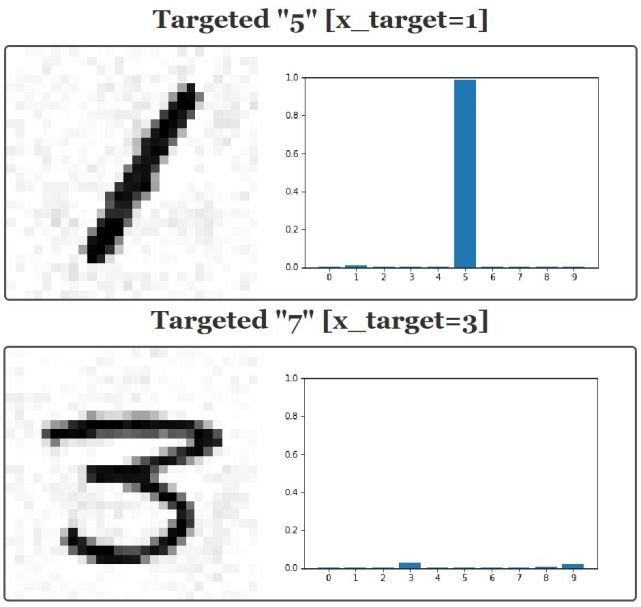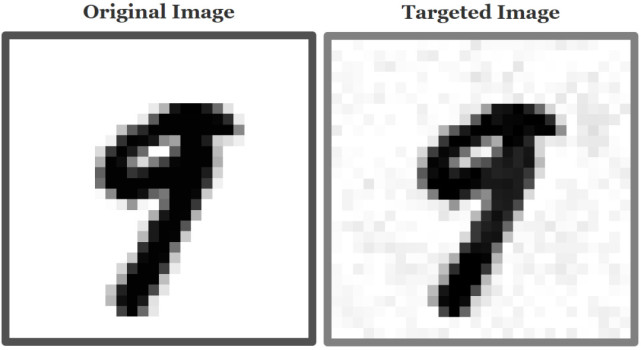```
def binary_thresholding(n, m): """ n: int 0-9, the target number to match m: index of example image to use (from the test set) """ # Generate adversarial example x = sneaky_generate(n, m) # Binarize image x = (x > .5).astype(float) print("With binary thresholding: ") plt.imshow(x.reshape(28,28), cmap="Greys") plt.show() # Get binarized output and prediction binary_activations = net.feedforward(x) binary_prediction = np.argmax(net.feedforward(x)) print("Prediction with binary thresholding: ") print(binary_prediction) print("Network output: ") print(binary_activations)

```